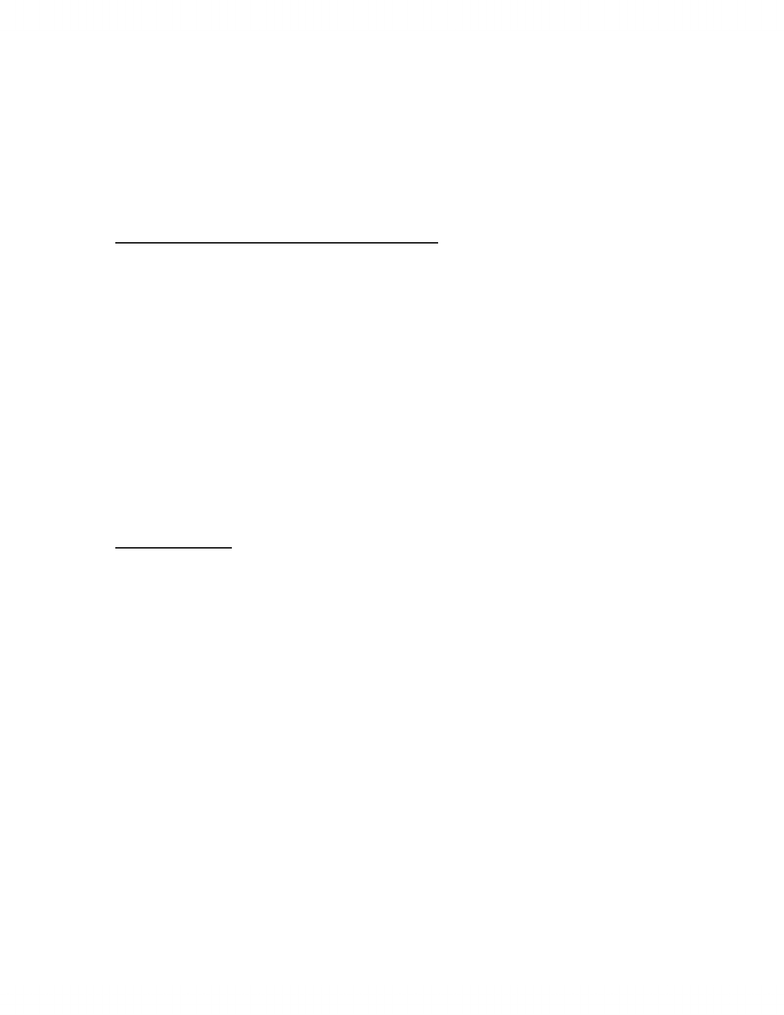# ACC410 Solution Chapter 3

111 views5 pages
School
Department
CourseChapter 3
Cost-Volume-Profit Analysis
MULTIPLE CHOICE QUESTIONS
3.14.
Ans: D
3.15.
Ans: B
3.16.
Ans: C
3.17.
Ans: A
3.18.
Ans: B
EXERCISES
3.19
B. Q = 74,667 child visits
3.21
A. V = \$31
B. F = \$4,664
C. Q = 360 units
3.22
A. Profit (loss) before taxes is = \$(100,000)
B. P = \$5.125
3.23
A. TC = \$38,363 + \$0.825*appointments
B. Q = 1,587 appointments
Unlock document

This preview shows pages 1-2 of the document.
Unlock all 5 pages and 3 million more documents.3-2 Cost Management
3.24
A. Q = 80,000 units
B. TR = \$10,000,000
C. TR = \$12,000,000
D. Q = 240,000 units
Dollar sales = \$12,000,000
3.25
A. Q = 15,000 units
B. Q = 29,000 units
C. Q = 19,125 units
D. Q = 31,625 units
E. P = \$28.33
3.26
A. TR = \$1,208,333.33
3.27
A. Q = 275,000 boxes
B. P = \$4.50
C. Dollar sales = \$1,920,000
3.28
A. Breakeven for option 1 = 400 sets
Breakeven for option 2 = 317 sets
Breakeven for option 3 = 0 sets
B. option 1
C. option 3
D. TR = \$18,000
E. Option 3 profit
3.29
A. ROI = 8.4%
B. TR = 2,410,500€
3.30
A. Q = 136,667 units.
B. 30,000 units, \$300,000
C. 3.33
Unlock document

This preview shows pages 1-2 of the document.
Unlock all 5 pages and 3 million more documents.